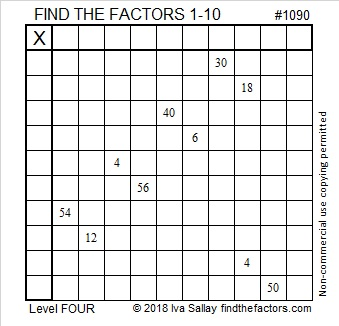# 1090 and Level 4

This puzzle has both 54 and 56 as clues. Many people get the factors involved (6, 7, 8, 9) mixed up. Remember these two multiplication facts:
6 7 × 8 9 = 54
6 7 × 8 9 = 56
The closer factors make the bigger number.Print the puzzles or type the solution in this excel file: 10-factors-1087-1094

Now I’ll share some information about the number 1090:

• 1090 is a composite number.
• Prime factorization: 1090 = 2 × 5 × 109
• The exponents in the prime factorization are 1, 1, and 1. Adding one to each and multiplying we get (1 + 1)(1 + 1)(1 + 1) = 2 × 2 × 2 = 8. Therefore 1090 has exactly 8 factors.
• Factors of 1090: 1, 2, 5, 10, 109, 218, 545, 1090
• Factor pairs: 1090 = 1 × 1090, 2 × 545, 5 × 218, or 10 × 109
• 1090 has no square factors that allow its square root to be simplified. √1090 ≈ 33.015151090 is the sum of the fourteen prime numbers from 47 to 107.

1090 is the sum of two squares two different ways:
27² + 19² = 1090
33² +  1² = 1090

1090 is the hypotenuse of four Pythagorean triples:
66-1088-1090 calculated from 2(33)(1), 33² –  1², 33² +  1²;
it is also 2 times (33-544-545),
368-1026-1090 calculated from 27² – 19² , 2(27)(19) , 27² + 19²;
it is also 2 times (184-513-545),
600-910-1090 which is 10 times (60-91-109)
654-872-1090 which is (3-4-5) times 218

1090 is a palindrome in two different bases:
It’s 1441 in BASE 9 because 1(9³) + 4(9²) + 4(9) + 1(1) = 1090
101 in BASE 33 because 33² + 1 = 1090

This site uses Akismet to reduce spam. Learn how your comment data is processed.